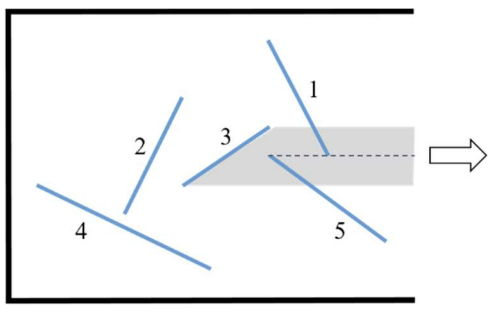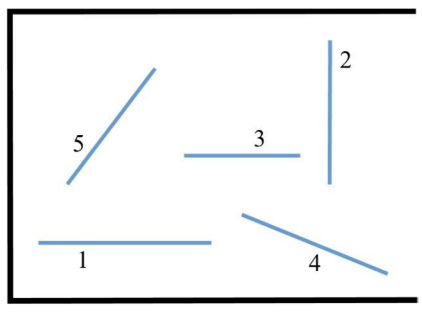시간 제한메모리 제한제출정답맞힌 사람정답 비율
0.5 초 1024 MB109281443.750%

## 문제

There are $n$ logs placed in a rectangular warehouse. The logs do not intersect or overlap each other. The right wall of the warehouse is open, through which logs can be dragged out to the right. A log is only moved parallel in the positive $x$-axis direction. A log can only be pulled out if there are no other logs in the space through which it will move. In Figure G.1, the movement space of log 3 is grayed out. Log 3 cannot be pulled out until logs 1 and 5 have been removed.

Multiple logs can be pulled out simultaneously if there are no other logs in the space through which they will move. Suppose that it takes 1 unit of time to pull out a log. Your task is to pull out all the logs of the warehouse as quickly as possible.

In Figure G.1, in order to pull out all five logs, you have to pull out the logs one by one in the order of 1-5-3- 2-4. Therefore, it takes 5 units of time to complete the task. Note that since the end point of log 1 is located in the movement space of log 5, it is not possible to pull out log 5 first.

Consider an example shown in Figure G.2. Logs 2 and 4 can be pulled out at the same time. After that, you can pull out logs 1 and 3 at the same time. Finally, you can pull out log 5. Therefore, it takes 3 units time.Figure G.1 Figure G.2

Given the locations of $n$ logs, write a program to find the minimum time required to pull out all the logs.

## 입력

Your program is to read from standard input. The input starts with a line containing an integer $n$ ($1 \le n \le 20,000$), where $n$ is the number of logs. The logs are numbered from $1$ to $n$. In the following $n$ lines, the $i$-th line contains four integers, $x_1$, $y_1$, $x_2$, and $y_2$, where $(x_1, y_1)$ and $(x_2, y_2)$ are the coordinates of both end points of the $i$-th log and all the integers are between $1$ and $10^9$. The length of a log is more than $0$ and no two logs intersect each other at any point.

## 출력

Your program is to write to standard output. Print exactly one line. The line should contain an integer representing the minimum units of time to pull out all the logs.

## 예제 입력 1

5
9 9 11 5
4 3 6 7
6 4 9 6
1 4 7 1
13 2 9 5


## 예제 출력 1

5


## 예제 입력 2

5
1 2 7 2
11 4 11 9
10 5 6 5
8 3 13 1
2 4 5 8


## 예제 출력 2

3